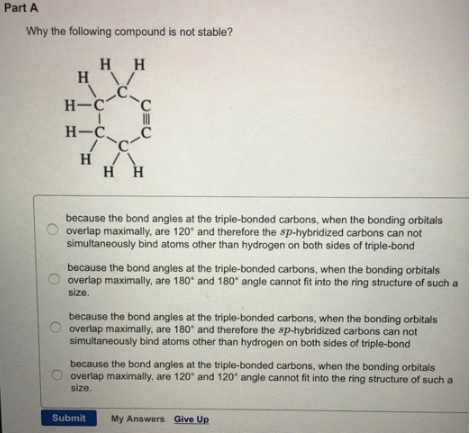# Problem: Why the following compound is not stable? (i) because the bond angles at the triple-bonded carbons, when the bonding orbitals overlap maximally, are 120° and therefore the sp-hybridized carbons can not simultaneously bind atoms other than hydrogen on both sides of triple-bond.(ii) because the bond angles at the triple-bonded carbons, when the bonding orbitals overlap maximally, are 180° and 180 degree angle cannot fit into the ring structure of such a size. (iii) because the bond angles at the triple-bonded carbons, when the bonding orbitals overlap maximally, are 180° and therefore the sp-hybridized carbons can not simultaneously bind atoms other than hydrogen on both sides of triple-bond.(iv) because the bond angles at the triple-bonded carbons, when the bonding orbitals overlap maximally, are 120° and 120° angle cannot fit into the ring structure of such a size.

###### FREE Expert Solution

Why the following compound is not stable?

(i) because the bond angles at the triple-bonded carbons, when the bonding orbitals overlap maximally, are 120° and therefore the sp-hybridized carbons can not simultaneously bind atoms other than hydrogen on both sides of triple-bond. (False, triple bonds (sp hybridized) follow linear geometry which follows an angle of 180°)

91% (72 ratings)###### Problem Details

Why the following compound is not stable?

(i) because the bond angles at the triple-bonded carbons, when the bonding orbitals overlap maximally, are 120° and therefore the sp-hybridized carbons can not simultaneously bind atoms other than hydrogen on both sides of triple-bond.

(ii) because the bond angles at the triple-bonded carbons, when the bonding orbitals overlap maximally, are 180° and 180 degree angle cannot fit into the ring structure of such a size.

(iii) because the bond angles at the triple-bonded carbons, when the bonding orbitals overlap maximally, are 180° and therefore the sp-hybridized carbons can not simultaneously bind atoms other than hydrogen on both sides of triple-bond.

(iv) because the bond angles at the triple-bonded carbons, when the bonding orbitals overlap maximally, are 120° and 120° angle cannot fit into the ring structure of such a size.